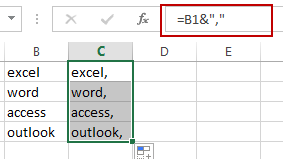# Adding Comma Character at End of Cells in Excel

This post will guide you how to add a comma character at end of cell or text in Excel. How do I add a comma after each cell with a formula in Excel. How to add comma to end of each cell in Excel.

## Adding Comma Character at End of Cells

If you want to add a comma character at end of cells in a range, you just need to use the concatenation operator to create a formula. Like this:

`=B1&","`

Type this formula in a blank cell, and then press Enter key. And then drag the AutoFill handle over other cells to apply this formula.You will see that the comma character has been added at the end of all cells.

Related Posts

Calculate Win Loss Tie

Suppose you got a task to calculate the win, loss, and tie totals; what would you do? If you are new to Ms Excel and don't have enough experience with it, then you might do this task manually but let ...

Calculate Years Between Dates In Ms Excel

If you are an avid Ms Excel user, then you might have come across a task in which you needed to calculate the years between the dates; you might take it easy and do this task manually, which is also ...

Calculate Number of Hours between Two Times

Calculating the difference between two times might be a valuable statistic for subsequent computations or averages, whether you're producing a time sheet for staff or recording personal exercises. While Excel has a plethora of complex functions, including date and time ...

Calculate Loan Interest in Given Year

When you borrow money, you are supposed to repay it gradually. Lenders, on the other hand, want to be compensated for their services and the risk they incur by lending you money. That is, you will not just repay the ...

Calculate Interest Rate for Loan

The interest rate is the fee charged by a lender to a borrower and is expressed as a percentage of the principal—the lent amount. The interest rate on a loan is often expressed as an annual percentage rate, abbreviated as ...

Sidebar